# GSEB Solutions Class 11 Maths Chapter 5 Complex Numbers and Quadratic Equations Miscellaneous Exercise

Gujarat Board GSEB Textbook Solutions Class 11 Maths Chapter 5 Complex Numbers and Quadratic Equations Miscellaneous Exercise Textbook Questions and Answers.

## Gujarat Board Textbook Solutions Class 11 Maths Chapter 5 Complex Numbers and Quadratic Equations Miscellaneous Exercise

Question 1.
Evaluate [i18 + ($$\frac{1}{i}$$)25]3.
Solution:
$$\frac{1}{i}$$ = $$\frac{1}{i}$$ × $$\frac{i}{i}$$ = $$\frac{i}{i^{2}}$$ = $$\frac{i}{- 1}$$ = – i.
∴ [i18 + ($$\frac{1}{i}$$)25]3 = [i18 + (- i)25]3 = [(i2)9 – i(i2)12]3
= [(- 1)9 – i( – 1)12]3
= [- 1 – i]3
= (- 1)3 + 3(- 1)2.(- i) + 3(- 1)(- i)2 + (- i)3
[∵ (a + b)3 = a3 + 3a2b + 3cb2 + b3]
= – 1 – 3i + 3 – i3
= – 1 – 3i + 3 – i(- 1)
= – 1 – 3i + 3 + i = 2 – 2i.Question 2.
For any two complex numbers z1 and z2, prove that Re(z1z2) = Rez1 Rez2 – Imz1 Imz2.
Solution:
Let z1 = a + ib, where a – Rez1; b = Im(Z1)
and z2 = c + id, where c – R(z2), d – Im(z2).
∴ z1z2 = (a + ib)(c + id) = ac – bd + i(ad + bc)
∴ Re(z1z2) = ac – bd
= Rez1. Rez2 – Imz1Imz2.

Question 3.
Reduce ($$\frac{1}{1 – 4i}$$ – $$\frac{2}{1 + i}$$) ($$\frac{3 – 4i}{5 + i}$$) to the standard form.
Solution: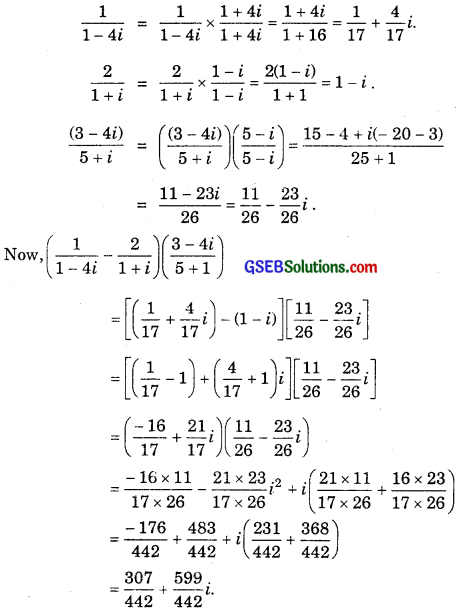Question 4.
If x – iy = $$\sqrt{\frac{a-i}{a+i b}}$$, prove that (x2 + y2)2 = $$\frac{a^{2}+b^{2}}{c^{2}+d^{2}}$$
Solution: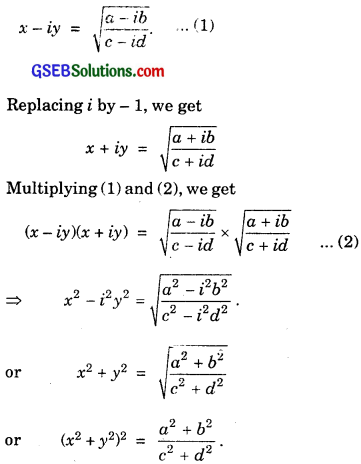Question 5.
Convert the following in the polar form:
(i) $$\frac{1+7 i}{(2-i)^{2}}$$
(ii) $$\frac{1 + 3i}{1 – 2i}$$
Solution: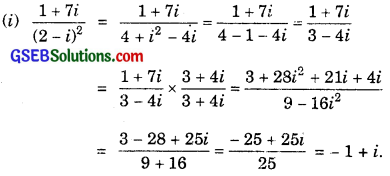Put rcos θ = – 1 and rsin θ = 1.
r2 = 1 + 1 = 2 ⇒ r = $$\sqrt{2}$$.
and cos θ = $$\frac{1}{\sqrt{2}}$$, sin θ = $$\frac{1}{\sqrt{2}}$$.
sin θ is + ve cos θ is – ve. Therefore, θ lies in II quadrant.
So, θ = π – $$\frac{π}{4}$$ = $$\frac{3π}{4}$$ [∵ sin θ = sin $$\frac{3π}{4}$$ = $$\frac{1}{\sqrt{2}}$$]
∴ Polar form of $$\frac{1+7 i}{(2-i)^{2}}$$ = $$\sqrt{2}$$(cos $$\frac{3π}{4}$$ + isin $$\frac{3π}{4}$$)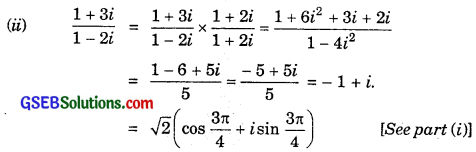Solve each of the equations in questions 6 to 9:
6. 3x2 – 4x + $$\frac{20}{3}$$ = 0
7. x2 – 2x + $$\frac{3}{2}$$ = 0
8. 27x2 – 10x + 1 = 0
9. 21x2 – 28x + 10 = 0
Solutions to questions 6 to 9:
6. 3x2 – 4x + $$\frac{20}{3}$$ = 0
Multiplying by 3, we get
9x2 – 12x + 20 = 0.
∴ a = 9, b = – 12, c = 20.
∴ b2 – 4ac = (- 12)2 – 4.9.20 = 144 – 720
= – 576.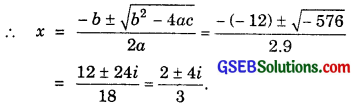7. x2 – 2x + $$\frac{3}{2}$$ = 0.
Multiplying by 2,
2x2 – 4x + 3 = 0.
Here, a = 2, b = – 4, c = 3
∴ b2 – 4ac = (- 4)2 – 4.2.3 = 16 – 24 = – 8.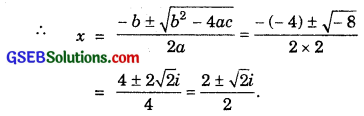8. 27x2 – 10x + 1 = 0
Here, a = 27, b = – 10, c = 1.
∴ b2 – 4ac = (- 10)2 – 4.27.1 = 100 – 108 = – 8.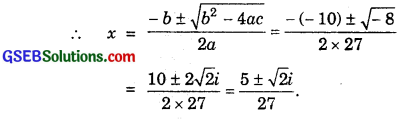9. 21x2 – 28x + 10 = 0.
Here, a = 21, b = – 28, c = 10
∴ b2 – 4ac = (- 28)2 – 4.21 × 10.
= 784 – 840 = – 56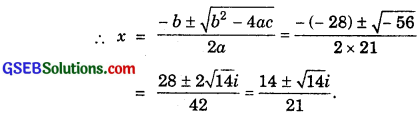10. If z1 = 2 – i and z2 = 1 + i, find |$$\frac{z_{1}+z_{2}+1}{z_{1}-z_{2}+i}$$|.
Solution: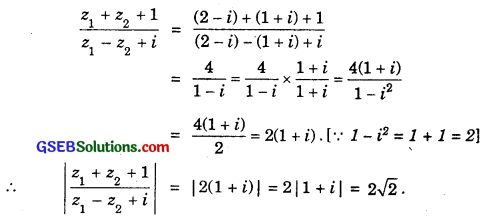11. If (a + b) = $$\frac{(x+i)^{2}}{2 x^{2}+1}$$, prove that a2 + b2 = $$\frac{\left(x^{2}+1\right)^{2}}{\left(2 x^{2}+1\right)^{2}}$$.
Solution:
(a + ib) = $$\frac{(x+i)^{2}}{2 x^{2}+1}$$ ………………… (1)
Replacing i by – i, we get
a – ib = $$\frac{(x-i)^{2}}{2 x^{2}+1}$$ …………………….. (2)
Multiplying (1) and (2), we get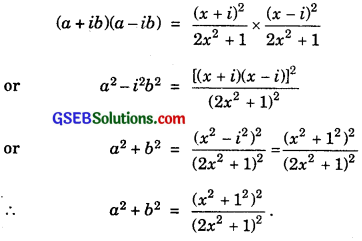12. Let z1 = 2 – i and z2 = – 2 + i. Find:
(i) Re$$\left(\frac{z_{1} z_{2}}{z_{1}}\right)$$
(ii) Im$$\left(\frac{1}{z z_{1}}\right)$$
Solution: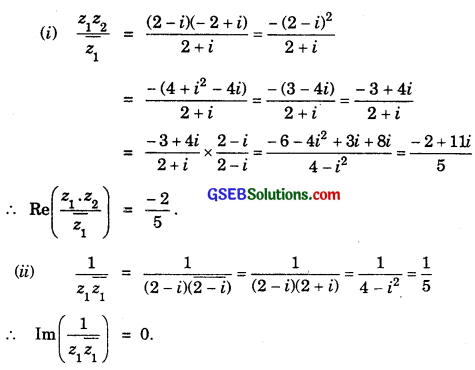13. Find the modulus and argument of the complex number $$\frac{1 + 2i}{1 – 3i}$$.
Solution: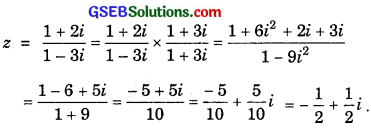Put r cos θ = – $$\frac{1}{2}$$ and r sin θ = $$\frac{1}{2}$$.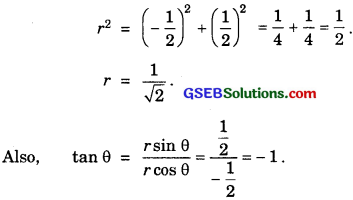sin θ is +ve and cos θ is -ve.
∴ θ = π – $$\frac{π}{4}$$ = $$\frac{3π}{4}$$.
∴ |$$\frac{1 + 2i}{1 – 3i}$$| = $$\frac{1}{\sqrt{2}}$$ and arg ($$\frac{1 + 2i}{1 – 3i}$$) = $$\frac{3π}{4}$$.14. Find the real number x and y, if (x – iy)(3 + 5i) is conjugate of – 6 – 24i.
Solution:
Conjugate of – 6 – 24i is – 6 + 24i …………………. (1)
Also, (x – iy)(3 + 5i) = 3x – 5yi2 – 3yi + 5xi
= (3x + 5y) + (5x – 3y)i …………………… (2)
Equating (1) and (2), we get
3x + 5y + (5x – 3y)i = – 6 + 24i
∴ 3x + 5y = – 6
and 5x – 3y = 24
or 3x + 5y + 6 = 0
and 5x – 3y – 24 = 0.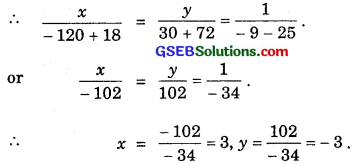∴ x = 3, y = – 3.

15. Find the modulus of $$\frac{1+i}{1-i}$$ = $$\frac{1-i}{1+i}$$.
Solution: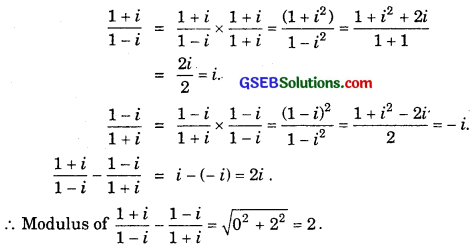16. If (x + iy)3 = u + iv, then show that $$\frac{u}{x}$$ + $$\frac{u}{y}$$ = 4(x2 – y2).
Solution:
(x + iy)3 = x3 + 3x2iy + 3x.(iy)2 + (iy)2
= x3 + 3x2yi – 3xy2 – iy3
∴ (x3 – 3xy2) + (3x2y – y3)i = u + iv.
Equating real and imaginary parts, we get
x3 – 3xy2 = u ∴ x2 – 3y2 = $$\frac{u}{x}$$ ……………….. (1)
3x2 – y3 = v ∴ 3x2 – y2 = $$\frac{v}{y}$$ ………………… (2)
Adding (1) and (2), 4x2 – 4y2 = $$\frac{u}{x}$$ + $$\frac{v}{y}$$
∴ $$\frac{u}{x}$$ + $$\frac{v}{y}$$ = 4(x2 – y2).17. If α and β are different complex numbers with |β| = 1, then find |$$\frac{\beta-\alpha}{1-\alpha \beta}$$|.
Solution: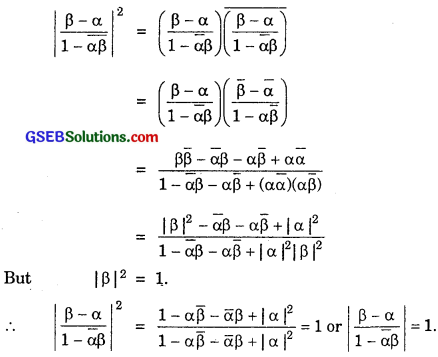18. Find the number of non-zero integral solutions of the equation |1 – i|x = 2x.
Solution:
|1 – i| = $$\sqrt{1^{2}+(-1)^{2}}$$ = $$\sqrt{2}$$ = 21/2.
∴ |1 – i|x = (21/2)x = 2x/2.
Since |1 – i|x = 2x, therefore
2x/2 = 2x.
∴ $$\frac{x}{2}$$ = x is admissible except x = 0.
⇒ There is no non-zero integral solution. Its solution is x = 0.

19. If (a + ib)(c + id)(e + if)(g + ih) = A + iB, then show that (a2 + b2)(c2 + d2)(c2 + f2) + (g2 + h2) = A2 + B2.
Solution:
(a + ib)(c + id)(e + if)(g + ih) = A + iB ………………. (1)
Replacing i by – i, we get
(a – ib)(c – id)(e – if)(g – ib) = A – iB …………………… (2)
Multiplying (1) and (2), we get
[(a + ib )(a – ib)] [(c + id)(c – id)] [(e + if)(e – if)] [(g + ih)(g – ih)] = (A + iB)(A – iB)
or (a2 – i2b2)(c2 – i2d2)(e2 – i2f2)(g2 – i2h2) = A2 – i2B2
(a2 + b2)(c2 + d2)(e2 + f2)(g2 + h2) = A2 + B2.
(a2 + b2)(c2 + d2)(e2 + f2)(g2 + h2) = A2 + B2. [∵ i2 = – 1]20. If ($$\frac{1+i}{1-i}$$)m = 1, then find the least integral value of m?
Solution: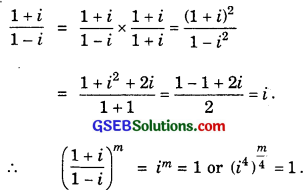⇒ m is a multiple of 4.
∴ Least value of m = 4.